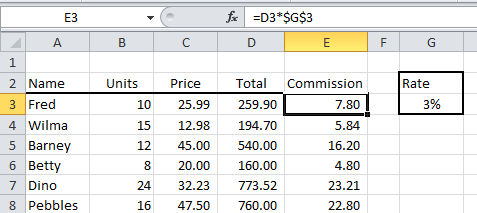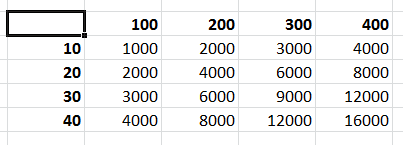## Tuesday, 21 October 2014

### Excel Absolute References, the Dollar sign \$ and the F4 key

Way back in the eighties we always used to say that if you didn't known your function keys then you weren't being serious. Thankfully those days have passed but if there's one function key you still really need to know for Excel formulas it's F4.

When you’re working in Word, Excel, or PowerPoint, the F4 key will repeat the last command or keystroke you just did. But it's primary use is for inserting the dollar signs (\$) for Absolute cell references in Excel formulas.

You may have seen cell references in formulas surrounded by ‘\$’ signs, for example \$D\$3:\$D\$10, and wondered what’s that all about?  The ‘\$’ before the column or row reference fixes the reference so that it does not change when it's copied. You either have to type in the \$ signs or press the F4 key.

For example, looking at the table below we have a Commission Rate of 3% in cell G3. In column E we want to calculate the commission as Total x Rate @ 3%.Calculating the commissions at 3%

We could simply enter the formula as =D3*3% and copy it down column E, but then that gives us two major problems to deal with:

1. We can’t easily see what the commission rate is without looking in the formula bar. We could include it in the column heading as "Commission @ 3%", but that makes the heading too wide and if I should change the rate then I will have to remember to go back and change the heading as well.
2. If I do change the rate then I need to change the formula and copy it down the column again. Once would be acceptable but several times not and what if other formulas are using the commission rate? It would be so much easier just to have the commission rate in a single cell.
In the formula for the commission calculation the commission rate of 3% is entered into cell G3 and then the G3 reference is used in our formulas like this, =D3*\$G\$3. The reference is absolute, meaning that it never changes wherever the formula is copied.

Let’s look at what happens if we don’t use an absolute reference. If we entered in cell E3 the formula =D3*G3 we would get the correct answer. But when we copy that formula down the rest of column E Excel updates the cell references in the formula to increase by one row as we go down. You can see this to the left where the references to D3 and G3 change to D4 and G4 etc.

These standard cell references are known as relative references. We want the D3 reference to change but we want the G3 to be fixed.

To keep the commission rate reference on cell G3 we enter the formula like this, =D3*\$G\$3. Then when we copy the formula down the column the column D references change but the reference to cell G3 does not.

Strictly speaking, we only needed to fix the reference to row 3 as the G column reference would not have changed but it's usually easier just to fix the entire cell reference and have done with it. The difficult bit is to realise that you needed an absolute reference in the first place.

### Other ways to use absolute references

• Make a whole range of cells an absolute reference: \$D\$1:\$F\$1
• Make only the column absolute \$D3
• Make only the row absolute D\$3
To help you see how your formulas are behaving it's quite a good idea if you can actually see them instead of the results of the formula. To display your formulas in the worksheet, click the Show Formulas control on the Formula Auditing group of the Formulas tab.

### Shortcut for entering Absolute References

The F4 key instantly enters the ‘\$’ signs for you. You can do it while you’re entering your formula or you can go back and edit the original formula.

In the example below we have started to enter a formula into cell E3. We have just selected cell G3, as you can see by the marquee ("marching ants") around the cell.Entering the formula

At this point, before pressing ENTER or clicking the tick to finish the formula, we can press the F4 key and Excel places the ‘\$’ signs around the G3 reference.Press F4 to enter the \$ signs

Or you can go back to a cell at any time. Press the F2 key to edit the cell if you are feeling old-fashioned or just double-click the cell. Click anywhere in the cell reference and press F4 to insert the \$ signs.If you want to fix a range reference you have to highlight the cell range in the formula before pressing F4. If you keep pressing F4 Excel iterates through all the permutations of absolute and relative reference:

• With the first press of F4 you get \$G\$3. Column and row absolute.
• With the second press of F4 you get G\$3. Column relative and row absolute.
• With the third press of F4 you get \$G3. Column absolute and row relative.
• With the fourth press of F4 you get G3. Column and row relative.

### Mixed Relative and Absolute references

Whilst it usually easier to fix the entire reference there are times when you must have a mixed reference, where only the row or the column is fixed, in order to make your formulas work. In the worksheet example below we are multiplying all the hundreds values in the top row against all the tens values in the first column of the table. The first formula multiplies the 10 by the 100, =B3*C2.Multiply the row value by the column values

As the formula is copied across and down we need the row reference for the hundreds values to be fixed and the column reference for the column reference for the tens values to be fixed. But the rest of the formula must be relative so that it works correctly when it is copied.Mixed Absolute and Relative references

Usually I try to work this type of formula out logically before I start. And usually it goes wrong so I just resort to experimentation until I get it right. It only takes me an hour or so.

#### Related Posts

1.Exactly what we needed. Thank you for making this available.

2.Wow, helps a lots, Thanks for it.

3.Amazing Blog...!! Thanks for sharing information. I will be waiting for next blog. Get to know more Advance Excel Training.

4.This content is written very well. Your use of formatting when making your points makes your observations very clear and easy to understand. Thank you.
excel vba courses london